# Integral equation

(diff) ← Older revision | Latest revision (diff) | Newer revision → (diff)

An equation containing the unknown function under the integral sign. Integral equations can be divided into two main classes: linear and non-linear integral equations (cf. also Linear integral equation; Non-linear integral equation).

Linear integral equations have the form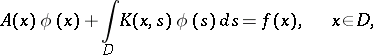(1)

where,,are given functions,being called the coefficient,the kernel (cf. Kernel of an integral operator) andthe free term (or right-hand side) of the integral equation,is a bounded or unbounded domain in a one- or higher-dimensional Euclidean space,are points of this space,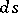is the volume element, andis the unknown function. It is required to determinesuch that (1) holds for all (or almost all, if the integral is taken in the sense of Lebesgue)in. In (1), if,are matrices and,are vector functions, then (1) is called a system of linear integral equations. If, then the integral equation is said to be homogeneous, otherwise it is called inhomogeneous.

There are three distinct types of linear integral equations, depending on the coefficient. If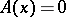for all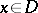, then (1) is called an equation of the first kind; iffor all, an equation of the second kind; and ifvanishes on some non-empty proper subset of, an equation of the third kind.

For simplicity, only integral equations in the one-dimensional case will be considered, whenis a finite interval. In this case, linear equations of the first and second kind can be represented in the following form:(2)(3)

respectively. The constantis called the parameter of the integral equation. Equations of the second kind are most frequently encountered in mathematical physics. Ifis a Fredholm kernel, that is, if the integral operator in equations (2), (3) is completely continuous (also called compact, see Completely-continuous operator), then the integral equation (2) (respectively, (3)) is called a Fredholm equation of the first (second) kind. An important example of a Fredholm equation is one in which the kernel satisfies the condition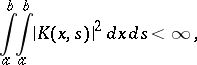(4)

while the right-hand side and the unknown functionare square-integrable functions.

The equation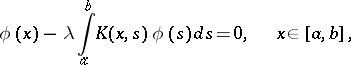(5)

is called the homogeneous integral equation corresponding to the inhomogeneous integral equation (3). The homogeneous integral equation corresponding to equation (2) is similarly defined. A homogeneous integral equation always has the solution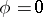, called the zero (or trivial) solution. The values of the parameterfor which (5) has a non-zero solutionare called the characteristic (or fundamental or eigen) values (or numbers) of the kernelor of the integral equation (5), while the non-zero solutionis called a characteristic (fundamental, eigen) function ofor of the integral equation (5), belonging (or corresponding) to the given eigen value. Ifis not an eigen value, then it is called a regular value (number).

The complex kernelis called Hermitian if(6)

where the bar denotes complex conjugation. In the case of a real kernel, (6) has the form. Such a kernel is called symmetric.

A Fredholm kernel need not have eigen values (for example, in the case of a Volterra kernel, see below). If the kernel is symmetric and does not vanish almost-everywhere, then it has at least one eigen value and all its eigen values are real.

If the kernelvanishes for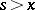(a so-called Volterra kernel), then equations (2) and (3) take the form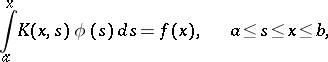(7)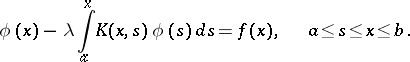(8)

These equations are called Volterra equations of the first and second kind, respectively (cf. Volterra equation). Special cases of integral equations began to appear in the first half of the 19th century. Integral equations became the object of special attention of mathematicians after the solution of the Dirichlet problem for the Laplace equation had been reduced to the study of a linear integral equation of the second kind. The construction of a general theory of linear integral equations was begun at the end of the 19th century. The founders of this theory are considered to be V. Volterra (1896), E. Fredholm (1903, ), D. Hilbert (1912, ), and E. Schmidt (1907, ). Even before these investigations, the method of successive approximation for the construction of a solution of an integral equation was proposed (cf. also Sequential approximation, method of). This method was initially applied to the solution of non-linear equations of Volterra type (in modern terminology) in connection with studies of ordinary differential equations in the work of J. Liouville (1838), L. Fuchs (1870), G. Peano (1888), and others; as well as by C. Neumann (1877) in constructing a solution of an integral equation of the second kind. The general form of the method of successive approximation is due to E. Picard (1893).

In studying the equation of a vibrating membrane H. Poincaré (1896) hit upon the idea of introducing a variable numerical parameterin equation (3). He then conjectured (by analogy with the case of a vibrating membrane) that the solution of (3) is a meromorphic function of. This conjecture was proved by Fredholm (1900–1903). The work of Fredholm was preceded by investigations of Volterra (1896–1897), who studied integral equations of the form (7), (8). He proved that if the kernel and the right-hand side are continuous, then for any finite value of, (8) has precisely one continuous solution, which can be constructed by the method of successive approximation. Equation (3) was studied by Fredholm under the assumption that its kernel, its right-hand side and the unknown solution are continuous functions on the squareand the interval, respectively. Following Volterra, Fredholm replaced the integral in (3) by a Riemann integral sum and considered the integral equation (3) as a limiting case of a finite system of linear algebraic equations (see Fredholm equation). By means of a formal limit transition Fredholm obtained a formula giving the solution to (3); he proved that this formula is a solution of (3) with the exception of a finite or countable set of values of the parameter, and proved theorems on the solvability of (3). Fredholm's theory for equation (3) was extended to the case of a system of integral equations and also to the case of a kernel with weak singularity (see Integral operator). The solution of a system reduces to that of a single equation, the kernel of which has lines of discontinuity parallel to coordinate axes.

Hilbert (1904) showed that the Fredholm theorems can be proved by a rigorous application of the process of limit transition and constructed a general theory of linear equations on the basis of the theory of linear and bilinear forms in an infinite number of variables. Schmidt

gave a simpler and somewhat more general version of the investigations of Hilbert. He constructed a theory of linear integral equations with real symmetric kernel (cf. Integral equation with symmetric kernel) independently of the Fredholm theory by representing the kernel as the sum of a degenerate and a "small" kernel. T. Carleman achieved a substantial weakening of the restrictions imposed on the data and the unknown elements in the theory of integral equations of the second kind for the case of real symmetric kernels. He extended the method of Fredholm (see ) to the case when the kernel of (3) satisfies condition (4). In papers of F. Riesz (1918) and J. Schauder (1930), Fredholm's theorems were generalized to a certain class of linear operator equations in Banach spaces.

The basic method for studying integral equations of the first kind is the so-called regularization method (see also Ill-posed problems). Integral equations of the third kind were the object of special investigations by H. Bateman (1907), Picard (1910), G. Fubini (1912), and Ch. Platrier (1912).

If a linear integral equation is not a Fredholm equation, then it is called a singular equation (cf. Singular integral equation). Hilbert's general theory of quadratic forms in an infinite number of variables provides the possibility in this case also of obtaining a number of important results. For certain specific classes of singular integral equations, special methods for solving them have been developed, taking into account the characteristic properties of these equations. For example, for singular integral equations and integral equations of convolution type (cf. Integral equation of convolution type), Fredholm's theorem that two transposed homogeneous integral equations have the same number of linearly independent solutions, is false.

In parallel with linear equations, non-linear integral equations have also been studied. In them, the unknown function can enter with power,, as, for example, in the equation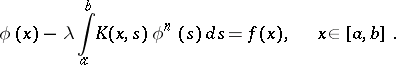It can also enter in a more general way, for example in the equation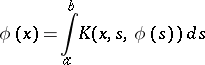How to Cite This Entry:
Integral equation. Encyclopedia of Mathematics. URL: http://encyclopediaofmath.org/index.php?title=Integral_equation&oldid=16255
This article was adapted from an original article by B.V. Khvedelidze (originator), which appeared in Encyclopedia of Mathematics - ISBN 1402006098. See original article